# RD Sharma Solutions for Class 9 Maths Chapter 1 Number System Exercise 1.2

RD Sharma Class 9 Mathematics Chapter 1 Exercise 1.2 Number System is provided here for students to prepare for exams. This set of solutions are designed by experts in accordance with CBSE latest syllabus, which covers decimal representation of rational numbers. Click on the link below to download free PDF of RD Sharma Solutions class 9 chapter 1.

## Download PDF of RD Sharma Solutions for Class 9 Maths Chapter 1 Number System Exercise 1.2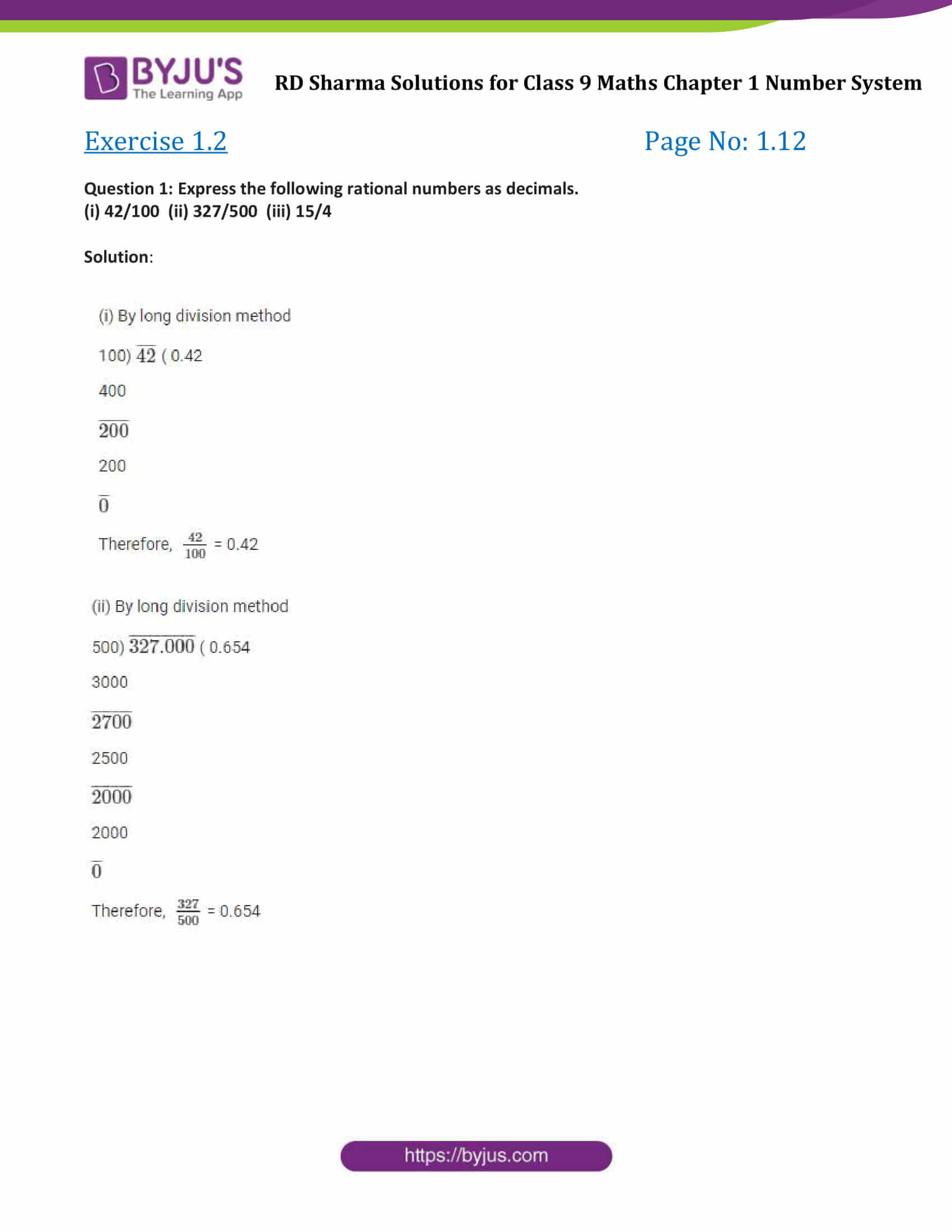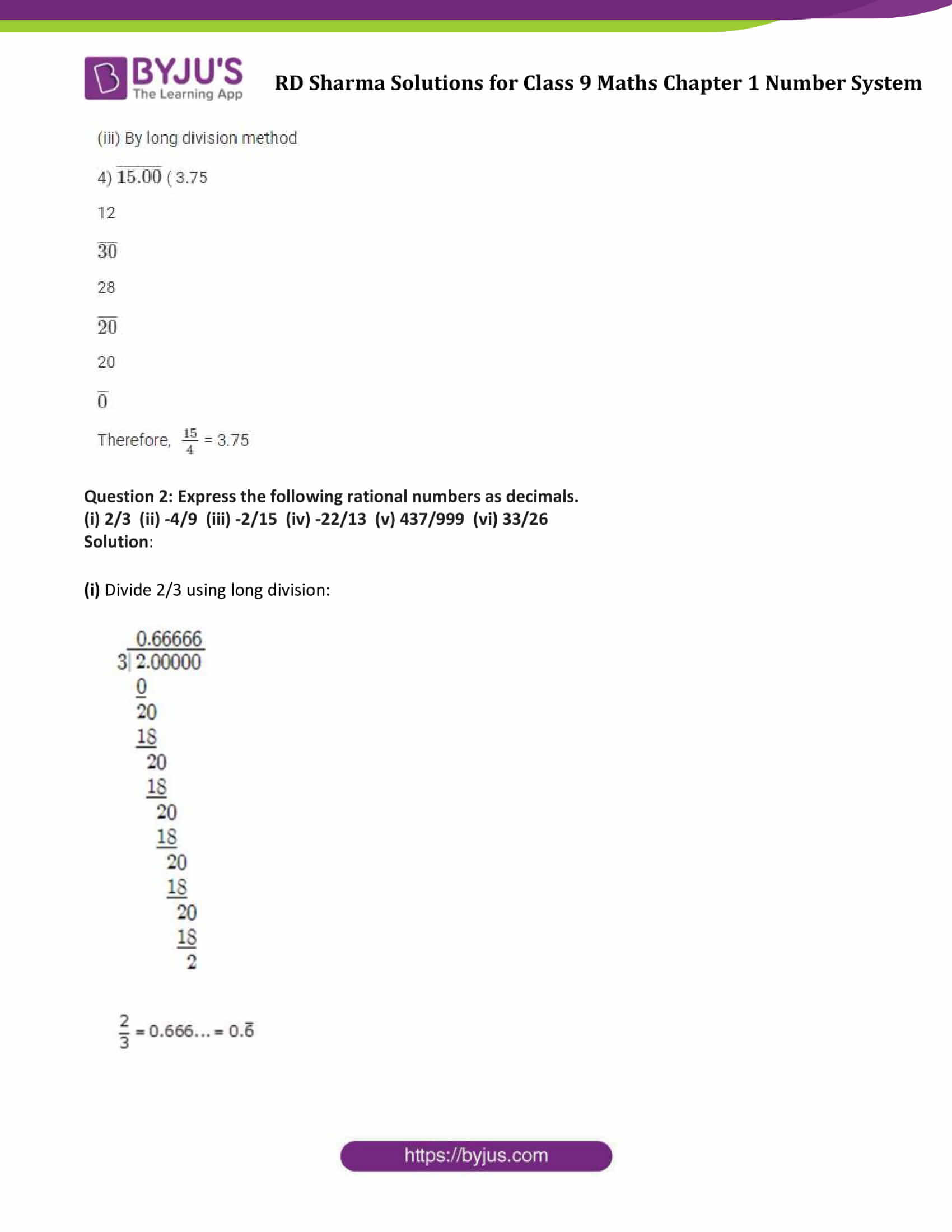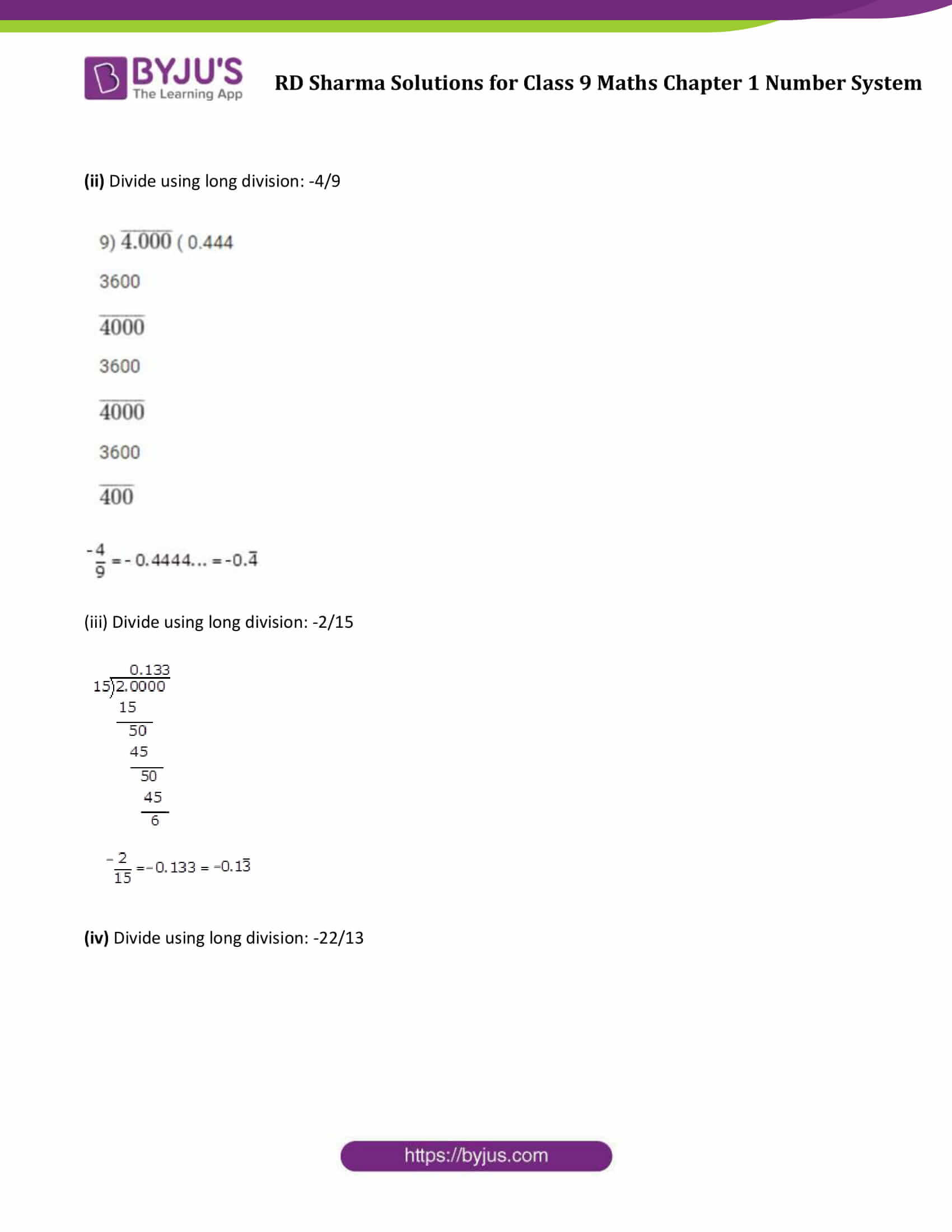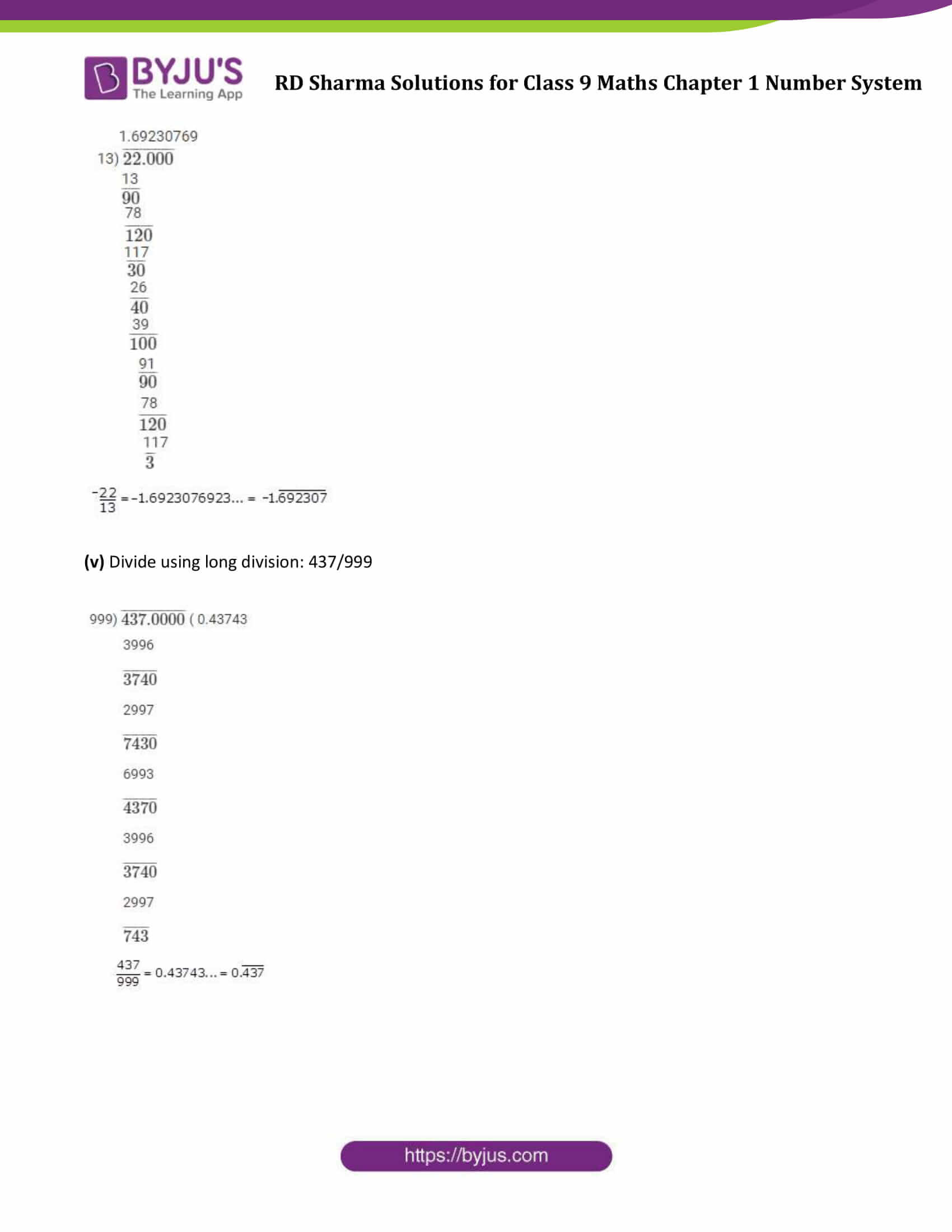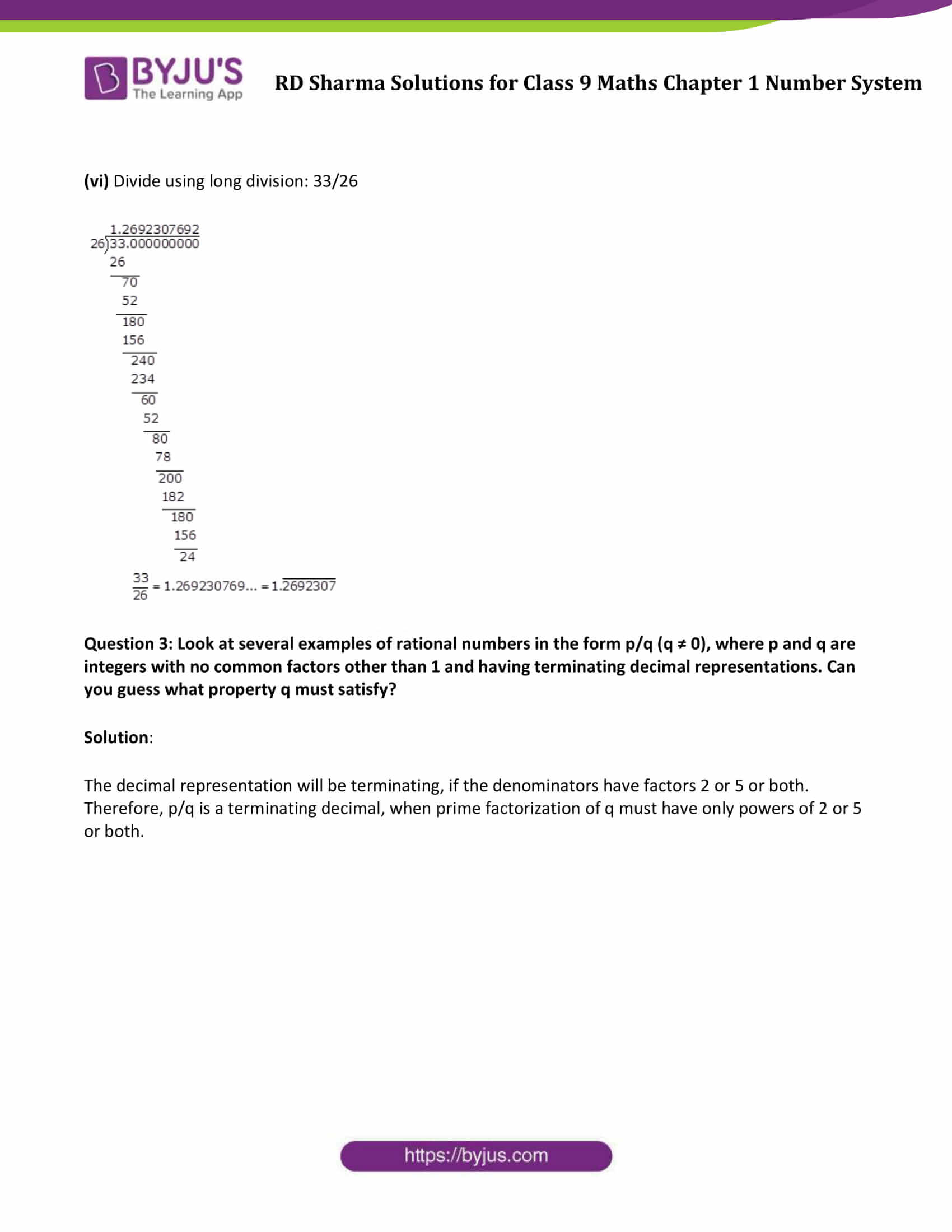### Access Answers to Maths RD Sharma Solutions for Class 9 Chapter 1 Number System Exercise 1.2 Page number 1.12

Question 1: Express the following rational numbers as decimals.
(i) 42/100 (ii) 327/500 (iii) 15/4

Solution: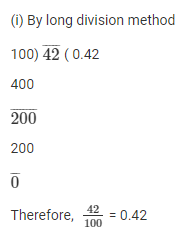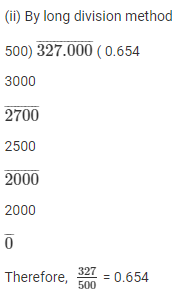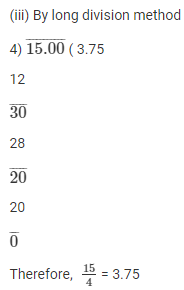Question 2: Express the following rational numbers as decimals.
(i) 2/3 (ii) -4/9 (iii) -2/15 (iv) -22/13 (v) 437/999 (vi) 33/26
Solution
:

(i) Divide 2/3 using long division: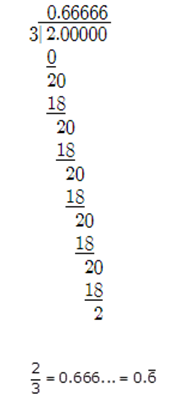(ii) Divide using long division: -4/9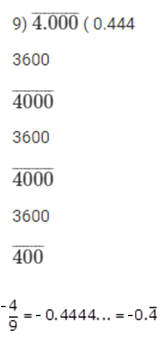(iii) Divide using long division: -2/15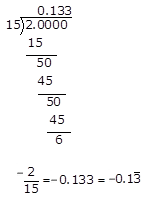(iv) Divide using long division: -22/13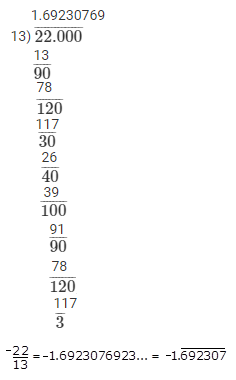(v) Divide using long division: 437/999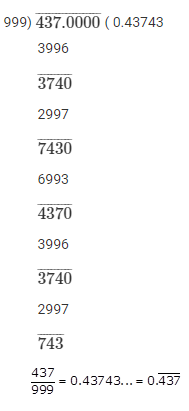(vi) Divide using long division: 33/26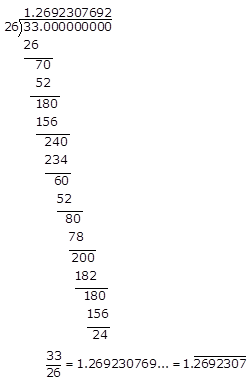Question 3: Look at several examples of rational numbers in the form p/q (q ≠ 0), where p and q are integers with no common factors other than 1 and having terminating decimal representations. Can you guess what property q must satisfy?

Solution:

The decimal representation will be terminating, if the denominators have factors 2 or 5 or both. Therefore, p/q is a terminating decimal, when prime factorization of q must have only powers of 2 or 5 or both.

## RD Sharma Solutions for Class 9 Maths Chapter 1 Number System Exercise 1.2

Class 9 Maths Chapter 1 Number System Exercise 1.2 is based on the decimal representation of rational numbers. To facilitate easy learning and help students understand the number system concepts, free RD Sharma Solutions Class 9 chapter 1 is provided here which can be further downloaded in the form of a PDF.